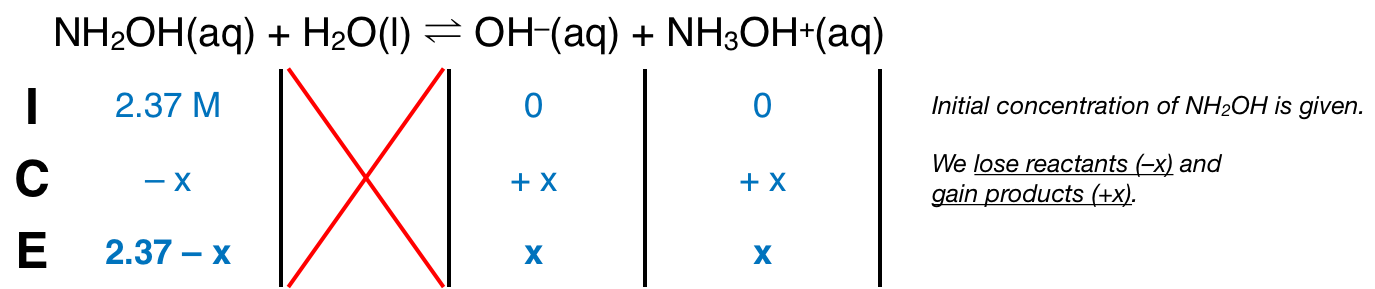# Problem: Hydroxylamine, NH2OH, is a weak base. The following is the equilibrium equation for its reaction with water:NH2OH(aq) + H2O(l) ⇌ NH3OH+(aq) + OH -       Kb = 9.1 x 10 -9What is the pOH of a 2.37 M NH 2OH solution?a) 1.15b) 3.83c) 4.99d) 6.72e) 8.13

###### FREE Expert Solution
83% (182 ratings)
###### FREE Expert Solution

We’re being asked to calculate the pOH of a 2.37 M NH2OH solution.

Since NH2OH has a low Kb value, it’s a weak base. Remember that weak bases partially dissociate in water and that bases accept H+ from the acid (water in this case). The dissociation of NH2OH is as follows:

NH2OH(aq) + H2O(l)  OH(aq) + NH3OH+(aq); Kb = 9.1 × 10–9

From this, we can construct an ICE table. Remember that liquids are ignored in the ICE table.The Kb expression for NH2OH is:Note that each concentration is raised by the stoichiometric coefficient: [NH2OH], [OH] and [NH3OH+] are raised to 1.

83% (182 ratings)###### Problem Details

Hydroxylamine, NH2OH, is a weak base. The following is the equilibrium equation for its reaction with water:

NH2OH(aq) + H2O(l) ⇌ NH3OH+(aq) + OH -       Kb = 9.1 x 10 -9

What is the pOH of a 2.37 M NH 2OH solution?

a) 1.15

b) 3.83

c) 4.99

d) 6.72

e) 8.13

What scientific concept do you need to know in order to solve this problem?

Our tutors have indicated that to solve this problem you will need to apply the Weak Bases concept. If you need more Weak Bases practice, you can also practice Weak Bases practice problems.

What is the difficulty of this problem?

Our tutors rated the difficulty ofHydroxylamine, NH2OH, is a weak base. The following is the e...as medium difficulty.

How long does this problem take to solve?

Our expert Chemistry tutor, Sabrina took 6 minutes and 46 seconds to solve this problem. You can follow their steps in the video explanation above.

What professor is this problem relevant for?

Based on our data, we think this problem is relevant for Professor Dunning's class at TEXAS.